## Selina Concise Mathematics Class 6 ICSE Solutions Chapter 11 Ratio

Selina Publishers Concise Mathematics Class 6 ICSE Solutions Chapter 11 Ratio

APlusTopper.com provides step by step solutions for Selina Concise ICSE Solutions for Class 6 Mathematics. You can download the Selina Concise Mathematics ICSE Solutions for Class 6 with Free PDF download option. Selina Publishers Concise Mathematics for Class 6 ICSE Solutions all questions are solved and explained by expert mathematic teachers as per ICSE board guidelines.

IMPORTANT POINTS

1. Ratio : The relation between two quantities (both of the same kind and in the same unit) obtained on dividing one quantity by the other, is called the ratio.
Keep in Mind :
(i) The ratio between two numbers or quantities is denoted by the colon “ : ”.
Thus, the ratio between two quantities p and q = p : q
(ii) The ratio between two quantities of same kind and in the same units is obtained on dividing one quantity by the other. Thus, the ratio between 20 kg and 80 kg = $$\frac { 20 }{ 80 } =\frac { 1 }{ 4 }$$ = 1 : 4
(iii) The first term of a ratio is called antecedent and its second term is called consequent. In the ratio 1:4; antecendent = 1 and consequent = 4.
(iv) A ratio must always by expressed in its lowest terms.
2. Proportion : A proportion is an expression which states that the two ratios are equal.
Keep in Mind :
In a proportion, its first and the fourth terms are called extremes whereas its second and the third terms are called means.
Thus, in 8 : 12 = 18 : 27; the terms 12 and 18 are means whereas 8 and 27 are extremes.
Also, Product of extremes = Product of Means

### Ratio Exercise 11A – Selina Concise Mathematics Class 6 ICSE Solutions

Question 1.
Express each of the following ratios in its simplest form :
(a) (i) 4 : 6
(ii) 48 : 54
(iii) 200 : 250
(b) (i) 5 kg : 800 gm
(ii) 30 cm : 2 m
(iii) 3 m : 90 cm
(iv) 2 years : 9 months
(v) 1 hour : 45 minutes
(vi) 4 min : 45 sec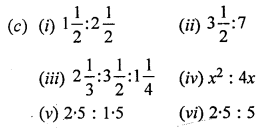Solution:Question 2.
A field is 80 m long and 60 m wide. Find the ratio of its width to its length.
Solution:
Width of field = 60 m
Length of field = 80 m
Ratio between width and length = $$\frac { 60 }{ 80 } =\frac { 3 }{ 4 }$$ = 3 : 4

Question 3.
State, true or false :
(i) A ratio equivalent to 7 : 9 is 27 : 21.
(ii) A ratio equivalent to 5 : 4 is 240 : 192.
(iii) A ratio of 250 gm and 3 kg is 1 : 12.
Solution:
(i) False.
Correct: A ratio equivalent to 7 : 9 is 9 : 7
(ii) True
(iii) True

Question 4.
Is the ratio of 15 kg and 35 kg same as the ratio of 6 years and 14 years ?
Solution: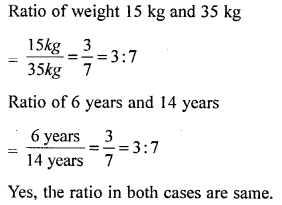Question 5.
Is the ratio of 6 g and 15 g same as the ratio of 36 cm and 90 cm ?
Solution:Question 6.
Find the ratio between 3.5 m, 475 cm and 2.8 m.
Solution:
The given values = 3.5 m, 475 cm and 2.8 m
= 3.5 x 100 cm : 475 cm : 2.8 x 100 cm
= 350 cm : 475 cm : 280 cm
= 70 cm : 95 cm : 56 cm
The ratio is 70 : 95 : 56

Question 7.
Find the ratio between 5 dozen and 2 scores. [1 score = 20]
Solution:
Ratio between 5 dozen and 2 scores
Given = 1 score = 20
then, 2 scores = 2 x 20 = 40
and 1 dozen = 12,
5 dozen = 12 x 5 = 60
Then, ratio = 60 : 40 = 3 : 2

### Ratio Exercise 11B – Selina Concise Mathematics Class 6 ICSE Solutions

Question 1.
The monthly salary of a person is Rs. 12,000 and his monthly expenditure is Rs 8,500. Find the ratio of his:
(i) salary to expenditure
(ii) expenditure to savings
(ii) savings to salary
Solution:
Monthly salary of a person = Rs 12,000
Monthly expenditure = 8,500
Saving of the person = (12,000 – 8500) = Rs 3,500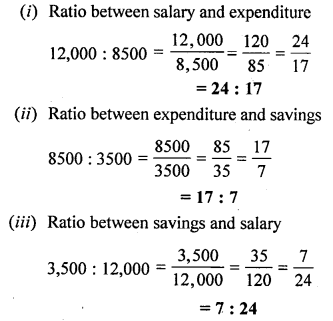Question 2.
The strength of a class is 65, including 30 girls. Find the ratio of the number of:
(i) girls to boys
(ii) boys to the whole class
(iii) the whole class to girls.
Solution:
Total strength of class (including boys and girls) = 65
Number of girls = 30
Number of boys = (65 – 30) = 35
Ratio between girls and boys = 30 : 35 = $$\frac { 30 }{ 35 } =\frac { 6 }{ 7 }$$ = 6 : 7Question 3.
The weekly expenses of a boy have increased from ₹ 1,500 to ₹ 2,250. Find the ratio of:
(i) increase in expenses to original expenses.
(ii) original expenses to increased expenses.
(iii) increased expenses to increase in expenses.
Solution:
Original expenses = ₹ 1500
Increased expenses = ₹ 2250
Increase in expenses = ₹ 2250 – ₹ 1500 = ₹ 750
Now,
(i) Ratio in increase in expenses to original expenses = ₹ 750 : ₹ 1500 = 1 : 2
(ii) Original expenses to increased expenses = ₹ 1500 : ₹ 2250
$$\frac { 1500 }{ 750 } =\frac { 2250 }{ 750 }$$ = 2 : 3
(iii) Increased expenses to increased in expenses = ₹ 2250 : ₹ 750 = 3 : 1 (Dividing by 750)

Question 4.
Reduce each of the following ratios to their lowest terms :
(i) 1 hour 20 min : 2 hours
(ii) 4 weeks : 49 days
(iii) 3 years 4 months : 5 years 5 months.
(iv) 2 m 40 cm : 1 m 44 cm
(v) 5 kg 500 gm : 2 kg 750 gm
Solution:
(i) 1 hour 20 min : 2 hour
= (1 x 60 + 20) minutes : 2 x 60 minutes
= 80 minutes : 120 minutes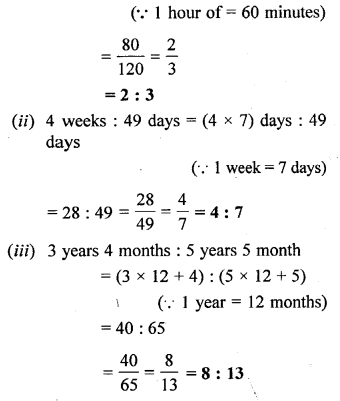Question 5.
Two numbers are in the ratio 9 : 2. If the smaller number is 320, find the larger number.
Solution:
Let the larger number = 9x
and smaller number = 2x
If smaller number is 320,
then, larger number will be = $$\frac { 9x\times 320 }{ 2x }$$ = 1440

Question 6.
A bus travels 180 km in 3 hours and a train travels 450 km in 5 hours. Find the ratio of speed of train to speed of bus.
Solution:
Distance travelled by bus = 180 km
Time taken = 3 hours
Speed = \frac { Distance }{ Time } =\frac { 180 }{ 3 } = 60 km/hr
Distance travelled by train = 450 km
Time taken = 5 hours
Speed = \frac { Distance }{ Time } =\frac { 450 }{ 5 } = 90 km/hr
Ratio of speed of train to speed of bus = 90 : 60 = 3 : 2

Question 7.
In winters, a school opens at 10 a.m. and closes at 3.30 p.m. If the lunch interval is of 30 minutes, find the ratio of lunch interval to total lime of the class periods.
Solution:
Timing of a school (10 a.m. to 3.30 p.m) = 5 hours 30 minutes
Timing for lunch interval = 30 minutes
Total time of the class periods = 5 hours 30 minutes – 30 minutes
= 5 hours = 60 x 5 = 300 minutes
Ratio of lunch interval to total time of the class period = 30 minutes : 300 minutes = 1 : 10

Question 8.
Rohit goes to school by car at 60 km per hour and Manoj goes to school by scooty at 40 km per hour. If they both live in the same locality, find the ratio between the time taken by Rohit and Manoj to reach school.
Solution:
Rohit travel by car, speed of the car = 60 km/hr
Manoj travel by scooty, speed of the scooty = 40 km/hr
Since, It is given that, they live in the same locality
Hence, let the distance be k Time taken by Rohit to reach school =Question 9.
In a club having 360 members, 40 play carrom, 96 play table tennis, 144 play badminton and remaining members play volley-ball. If no member plays two or more games, find the ratio of members who play :
(i) carrom to the number of those who play badminton.
(ii) badminton to the number of those who play table-tennis.
(iii) table-tennis to the number of those who play volley-ball.
(iv) volleyball to the n umber of those who play other games.
Solution:
Total members in a club = 360 members
Members who play carrom = 40
Members who play table tennis = 96
Members who play badminton = 144
Members who play volleyball = 360 – (40 + 96 + 144) = 360 – 280 = 80
(i) Ratio between the members who play carrom to the number of those who play badminton = 40 : 144 => 5 : 18
(ii) Ratio of members who play badminton to the number of those who play table- tennis = 144 : 96 => 6 : 4 = 3 : 2
(iii) Ratio of members who play table tennis to the number of those who play volley-ball = 96 : 80 = 6 : 5
(iv) Ratio of members who play volley-ball to the number of those who play other games = 80 : 280 =>4 : 14 = 2 : 7

Question 10.
The length of a pencil is 18 cm and its radius is 4 cm. Find the ratio of its length to its diameter.
Solution:
Length of a pencil = 18 cm
Radius of a pencil = 4 cm
Diameter of a pencil = 2r = 2 x 4 = 8 cm
Ratio of length of a pencil to its diameter = 18 : 8 = 19 : 2

Question 11.
Ratio of distance of the school from A’s home to the distance of the school from B’s home is 2 : 1.
(i) Who lives nearer to the school ?
(ii) Complete the following table :

Solution:
Ratio of distance of the school from A’s home to the distance of the school from B’s hence = 2 : 1
(i) B lives nearest to the school
(ii) Let A’s home is 2x km from school and B’s home is x km
Distance of school from A’s home = 2 x Distance of school from B’s home
=> If A lives at a distance of 4 km, then B lives at a distance of = $$\frac { 1 }{ 2 }$$ x 4 = 2 km
=> If B lives at a distance of 9 km then A lives at a distance of = 2 x 9 = 18 km
=> If A lives at a distance of 8 km then B lives at a distance = $$\frac { 1 }{ 2 }$$ x 8 = 4 km
=> If B lives at a distance of 8 km, then A lives at a distance = 2 x 8 = 16 km
=> If A lives at a distance of 6 km, then B lives at a distance = $$\frac { 1 }{ 2 }$$ x 6 = 3 km

Question 12.
The student-teacher ratio in a school is 45 : 2. If there are 4050 students in the school, how many teachers must be there?
Solution:
Total number of students = 4050
Let total number of teachers = x
The student-teacher ratio in a school = 45 : 2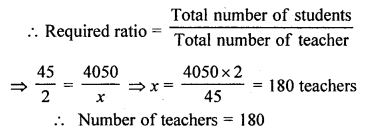### Ratio Exercise 11C – Selina Concise Mathematics Class 6 ICSE Solutions

Question 1.
₹ 120 is to be divided between Hari and Gopi in the ratio 5 : 3. How much does each get ?
Solution: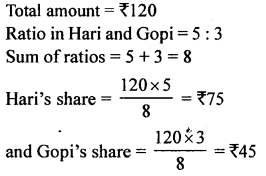Question 2.
Divide 72 in the ratio $$2\frac { 1 }{ 2 } :1\frac { 1 }{ 2 }$$
Solution: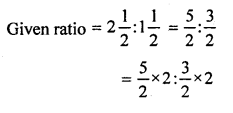Question 3.
Divide 81 into three parts in the ratio 2 : 3 : 4.
Solution:
Given ratio = 2 : 3 : 4
Sum of ratio = 2 + 3 + 4 = 9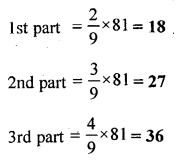Question 4.
Divide Rs 10,400 among A, B and C in the ratio $$\frac { 1 }{ 2 } :\frac { 1 }{ 3 } :\frac { 1 }{ 4 }$$
Solution:Question 5.
A profit of Rs 2,500 is to be shared among three persons in the ratio 6 : 9 : 10. How much does each person get?
Solution:
Total profit = Rs 2,500
Given ratio = 6 : 9 : 10
Sum of ratio = 6 + 9 + 10 = 25Question 6.
The angles of a triangle are in the ratio 3 : 7 : 8. Find the greatest and the smallest angles.
Solution:
Sum of angles of triangle = 180°
Given ratio 3 : 7 : 8
Sum of ratio = 3 + 7 + 8= 18
Smallest angle = $$\frac { 3 }{ 18 }$$ x 180° = 30°
Greatest angle = $$\frac { 8 }{ 18 }$$ x 180° = 80°

Question 7.
The sides of a triangle are in the ratio 3 : 2 : 4. If the perimeter of the triangle is 27 cm, find the length of each side.
Solution:
Ratio in the sides of a triangle = 3 : 2 : 4
Sum of ratios = 3 + 2 + 4 = 9
Perimeter of triangle = 27 cm
Length of first side = $$\frac { 27\times 3 }{ 9 }$$ = 9 cm
Length of second side = $$\frac { 27\times 2 }{ 9 }$$ = 6 cm
Length of third side = $$\frac { 27\times 4 }{ 9 }$$ = 12 cm

Question 8.
An alloy of zinc and copper weighs 12$$\frac { 1 }{ 2 }$$ kg. if in the alloy, the ratio of zinc and copper is 1 : 4, find the weight of copper in it.
Solution:
Weight of alloy = 12$$\frac { 1 }{ 2 }$$ kg = $$\frac { 25 }{ 2 }$$ kg.
Given ratio =1 : 4
Sum of ratio =1 + 4 = 5
Weight of copper = $$\frac { 4 }{ 5 } \times \frac { 25 }{ 2 }$$ kg = 2 x 5 = 10 kg

Question 9.
How will Rs 31500 be shared between A, B and C ; if A gets the double of what B gets, and B gets the double of what C gets ?
Solution:
Let the share of C = 1
Share of B = double of C = 2 x 1 = 2
Share of A = double of B = 2 x 2 = 4
Given ratio (A : B : C) = 4 : 2 : 1
Sum of ratio = 4 + 2 + 1 = 7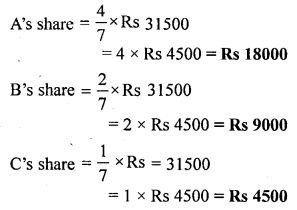Question 10.
Mr. Gupta divides Rs 81000 among his three children Ashok, Mohit and Geeta in such a way that Ashok gets four times what Mohit gets and Mohit gets 2.5 times what Geeta gets. Find the share of each of them.
Solution:
Let the share of Geeta = 1
Share of Mohit is (2-5 times of Geeta) = 2.5
Share of Ashok is (4 times of Mohit) = 4 x 2.5 = 10
Ratio = 1 : 2.5 : 10 = 1 x 2 :2.5 x 2 : 10 x 2 = 2 : 5 : 20
Sum of ratio = 2 + 5 + 20 = 27
Share of Geeta = $$\frac { 2 }{ 27 }$$ x Rs 81000 = 2 x Rs 3000 = Rs 6000
Share of Mohit = $$\frac { 5 }{ 27 }$$ x Rs 81000 = 5 x Rs 3000 = Rs 15000
Share of Ashok = $$\frac { 20 }{ 27 }$$ x Rs 81000 = 20 x Rs 3000 = Rs 60000

### Ratio Exercise 11D – Selina Concise Mathematics Class 6 ICSE Solutions

Question 1.
Which ratio is greater:Solution:Question 2.
Which ratio is smaller :Solution:Question 3.
Increase 95 in the ratio 5 : 8.
Solution:
Given ratio = 5 : 8
The increased quantity = $$\frac { 8 }{ 5 }$$ x given quantity
= $$\frac { 8 }{ 5 }$$ x 95 = 152

Question 4.
Decrease 275 in the ratio 11 : 7.
Solution:
Given ratio = 11 : 7
The decrease quantity= $$\frac { 11 }{ 7 }$$ x given quantity.
= $$\frac { 11 }{ 7 }$$ x 275 = 175

Question 5.
Decrease 850 in the ratio 17 : 6 and then increase the result in the ratio 5 : 9.
Solution:
The given quantity = 850 and decrease in the ratio =17 : 6
The decreased quantity = $$\frac { 6 }{ 17 }$$ x 850 = 300
Now, the quantity is increased in the ratio 5 : 9
The resulting quantity = $$\frac { 9 }{ 5 }$$ x 300 = 540

Question 6.
Decrease 850 in the ratio 17 : 6 and then decrease the resulting number again in 4 : 3.
Solution:
The given quantity = 850 and decrease in the ratio = 17:6
The decreased quantity = $$\frac { 6 }{ 17 }$$ x 850 = 300
Now, the quantity is decreased again in the ratio = 4 : 3
The resulting quantity = $$\frac { 3 }{ 4 }$$ x 300 = 225

Question 7.
Increase 1200 in the ratio 2 : 3 and then decrease the resulting number in the ratio 10 : 3.
Solution:
The given quantity = 1200 and increase in the ratio is 2 : 3
The increased quantity = $$\frac { 3 }{ 2 }$$ x 1200 = 1800
Now, the quantity is decrease in the ratio 10 : 3
= $$\frac { 3 }{ 10 }$$ x 1800 = 540

Question 8.
Increase 1200 in the ratio 3 : 7 and then increase the resulting number again in the ratio 4 : 7.
Solution:
The given quantity = 1200 increase in the ratio is 3 : 7
The increased quantity = $$\frac { 7 }{ 3 }$$ x 1200 = 2800
Now, the quantity is increased again in the ratio 4 : 7
The resulting quantity = $$\frac { 7 }{ 4 }$$ x 2800 = 4900

Question 9.
The number 650 is decreased to 500 in the ratio a : b, find the ratio a : b.
Solution:
The given quantity = 650
and the decreased quantity = 500
The ratio (a : b) by which 650 is decreased to 500 = $$\frac { 650 }{ 500 } =\frac { 13 }{ 10 }$$
The resulting ratio =13 : 10

Question 10.
The number 800 is increased to 960 in the ratio a : b, find the ratio a : b.
Solution:
The given quantity = 800
and the increased quantity = 960
The ratio (a : b) by which 800 is increased to 960 = $$\frac { 800 }{ 960 } =\frac { 5 }{ 6 }$$
The resulting ratio = 5 : 6